# Kerala Syllabus 8th Standard Basic Science Solutions Chapter 18 Reflection of Light in Spherical Mirrors

You can Download Reflection of Light in Spherical Mirrors Questions and Answers, Summary, Activity, Notes, Kerala Syllabus 8th Standard Basic Science Solutions Chapter 18 help you to revise complete Syllabus and score more marks in your examinations.

## Kerala State Syllabus 8th Standard Basic Science Solutions Chapter 18 Reflection of Light in Spherical Mirrors

The phenomena of light is always a surprising one. From long time ago man has tried to study about light. This chapter explains to draw the figure of the images formed by mirrors, their uses, magnification etc.

Spherical mirrors

Images are formed not only in-plane mirrors but also in smooth curved surfaces. Spherical mirrors are mirrors in which the reflecting surface is a part of the sphere. The center of a sphere of which the mirror is a part is the center of curvature. Any I line drawn from the center of curvature to the mirror is normal to the mirror. Radius of curvature of a mirror is the radius of the sphere of which is a part. The reflecting surface of the mirror is called the aperture of a mirror. The midpoint of the reflecting surface is called the pole. The straight line connecting the pole and center of curvature of a mirror is the principal axis of the mirror.

The angle of incident and angle of reflection are equal in spherical mirrors. Rays of light incident on a concave mirror, parallel to the principal axis, passes through a fixed point on the principal axis after reflection. This point is the principal focus of the concave mirror. Rays of light incident on a convex mirror parallel to the principal axis appear to come from a fixed point on the other side of the mirror. The point is the principal axis of the convex mirror.

Focal length of a mirror is the distance from pole of the mirror and the principal’s focus of the mirror. Rays of light coming from infinity get focused on a plane perpendicular to the principal axis. This plane is the focus plane. The focus plane passes through the focus plane.

Images formed by spherical mirrors

The image of an object placed different positions in front of a mirror is.formed in different positions. Rays of light incident on a concave mirror, parallel to the principal axis, passes through a fixed point on the principal axis after reflection Rays incident on an a concave mirror through the principal focus are reflected parallel to the principal axis.

Rays incident through the center of curvature reflected through the same path. Rays incident on the pole makes an angle with the principal axis. The ray diagrams of images formed by spherical mirrors are drawn according to the above rules. An object placed between F and C in front of a concave mirror, the image will be real and inverted. If the object is at F the image is formed at infinity. The paths of reflected rays are parallel to each other. If the object is in between F and P the image is formed behind the mirror. The image is erect and virtual.Magnification

Magnification is the ratio of the height of the image to the height of the object.

Uses of spherical mirrors

Spherical mirrors are used in lighthouses and reflectors.

### Reflection of Light in Spherical Mirrors Textbook Questions and Answers

Uses of Concave Mirror Questions 1.
Classify the following statements as to those related to concave mirrors and convex mirrors and tabulate them accordingly.
a. to view the face
b. as makeup mirror
c. as rearview mirrors in vehicles
d. in solar concentrators
e. in periscopes
f. as shaving mirror
Concave mirror:

• In solar concentrators
• Makeup mirror
• Shaving mirror

Convex mirror:

• In rearview mirrors of vehicles
• In Searchlights

Plane mirror:

• In periscopes
• To see face

Shaving Mirror Question 2.
Calculate the radius of curvature of a convex mirror of focal length 12 cm.
focal length of the mirror = 12 cm
$$\mathrm{f}=\frac{\mathrm{R}}{2} \quad 12=\frac{\mathrm{R}}{2}$$
R = 2 × 12 = 24 cm

Magnified Makeup Mirror Question 3.
A ray of light is made to fall on the pole of a concave mirror making an angle 30° with the principal axis.
a. What is the angle of reflection?
c. Draw the ray diagram.
a. Angle of reflection is 30°
b. angle of incidence and angle of reflection are equal
c. the figure showing angle of incidence and angle of reflection is 210°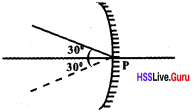Concave Mirror Question 4.
Which type of mirror always gives an erect and diminished image?
Convex mirror

Spherical Mirror Question 5.
A ray of light incident on a spherical mirror gets reflected along the same path. If so, show the light incident on the mirror.Question 6.
OA is a ray of light incident on a concave mirror.
a. Draw the path of the reflected ray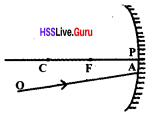b. On what basis did you mark the reflected ray?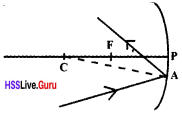b. In mirrors angle of incidence and angle of reflection are same. The normal to the point of incidence is passed through the center of curva¬ture. The angle between ray of reflection and the normal is the same as angle of incidence.

Reflection of Light by Spherical Mirrors Question 7.
Write down the type of mirrors that should be used for getting the following type of images.
a. real and magnified
b. virtual and magnified
c. virtual and diminished
d. real and diminished
a. concave mirror
b. concave mirror
c. convex mirror
d. concave mirror

Question 8.
The height of an object kept 12 cm away from a concave mirror is 1 cm. Calculate the magnifica¬tion if an image of height 2.5 cm is formed in front of the mirror.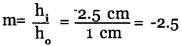Spherical Mirrors Image formation Question 9.
Which type of mirror always gives a virtual and erect-image, b. Is this image magnified or diminished?
a. convex mirror
b. Diminished

Concave & Convex Mirrors Question 1.
Complete the table

 No Angle of incidence Angle of reflection 1 30° …… (a) ……. 2 …. (b)… 40° 3 50° …. (c)… 4 60° … (d) ….

a. 30°
b. 40°
c. 50°
d. 60°

Question 2.
If the radius of curvature of a concave mirror is 24 cm what is its focal length?
R = 24 cmQuestion 3.
Find the radius of curvature of a convex mirror of focal length 0.6 mQuestion 4.
Write the characteristics of the image formed by an object plac¬ed at the center of curvature of a concave mirror?
Position: At the center of curvature at the same side
Size : Same as the size of the object Nature: Real, Inverted

Question 5.
Complete the figureQuestion 6.
Complete the table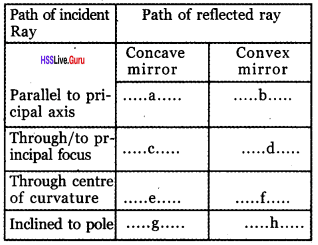a. Passes through principal focus
b. Seem to come from the principal focus
c, d. Returns parallel to the principal axis
e, f. Returns through the same path
g, h. Reflects in the same angle of incident ray.

Question 7.
Write three differences of real image and virtual image which is made by spherical mirrors
Real image:

1. Inverted
2. can be shown on the screen
3. can measure the length of the image and distance to the image

Virtual image:

1. Cannot show on the screen
2. Cannot measure
3. Erect

Question 8.
Write uses of concave mirrors

•  As shaving mirror
• As makeup mirror
• As head mirrors used by doctors
• In film projectors

Question 9.
Examine the position off the object given in the figure and table the following peculiarities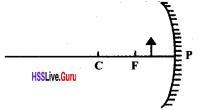a. position of the image
b. size of the image
c. nature of the image
a. Behind the mirror.
b. Larger than the object.
c. Erect and virtual

Question 10.
When an object of height 4 cm is placed in front of a concave mirror an image of height 8cm is formed. Find magnification.
hi = 4cm ho= -8 cm
Magnification = $$\frac{h_{i}}{h_{0}}$$ = $$\frac{h_{-8}}{h_{4}}$$ = -2Question 11.a. Examine the figure and find the magnification
b. What is the height of the object if height of the image is 4cm when the object is placed on the same position in front of the mirror.